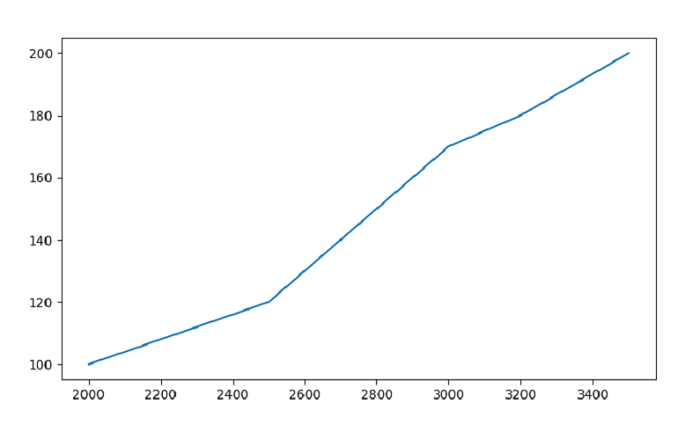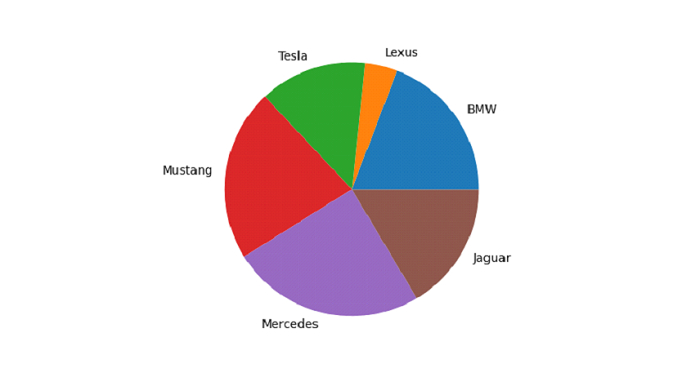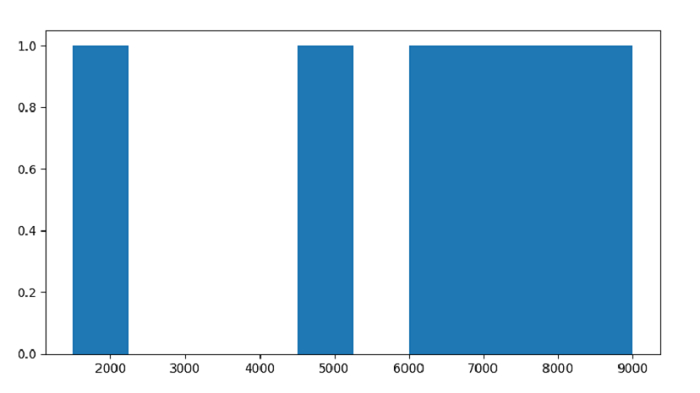# How to plot a Pandas Dataframe with Matplotlib?

We can plot Line Graph, Pie Chart, Histogram, etc. with a Pandas DataFrame using Matplotlib. For this, we need to import Pandas and Matplotlib libraries −

import pandas as pd
import matplotlib.pyplot as plt

Let us begin plotting −

## Line Graph

### Example

Following is the code −

import pandas as pd
import matplotlib.pyplot as plt

# creating a DataFrame with 2 columns
dataFrame = pd.DataFrame(
{
"Car": ['BMW', 'Lexus', 'Audi', 'Mustang', 'Bentley', 'Jaguar'],
"Reg_Price": [2000, 2500, 2800, 3000, 3200, 3500],
"Units": [100, 120, 150, 170, 180, 200]
}
)

# plot a line graph
plt.plot(dataFrame["Reg_Price"], dataFrame["Units"])
plt.show()

## Output

This will produce the following output −## Pie Chart

### Example

Following is the code to plot a Pie Chart −

import pandas as pd
import matplotlib.pyplot as plt

# creating dataframe
dataFrame = pd.DataFrame({
"Car": ['BMW', 'Lexus', 'Tesla', 'Mustang', 'Mercedes', 'Jaguar'],
"Reg_Price": [7000, 1500, 5000, 8000, 9000, 6000]
})

# plot a Pie Chart for Registration Price column with label Car column
plt.pie(dataFrame["Reg_Price"], labels = dataFrame["Car"])
plt.show()

## Output

This will produce the following output −## Histogram

### Example

Following is the code to plot Histogram −

import pandas as pd
import matplotlib.pyplot as plt

# creating dataframe
dataFrame = pd.DataFrame({
"Car": ['BMW', 'Lexus', 'Tesla', 'Mustang', 'Mercedes', 'Jaguar'],"Reg_Price": [7000, 1500, 5000, 8000, 9000, 6000]
})

# plot a histogram for Registration Price column
plt.hist(dataFrame["Reg_Price"])
plt.show()

## Output

This will produce the following output −Updated on: 30-Sep-2021

784 Views